# Christoffel symbol

(diff) ← Older revision | Latest revision (diff) | Newer revision → (diff)
Jump to: navigation, search

of a differential quadratic formAn abbreviated notation for the expressionThe symbols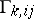are called the Christoffel symbols of the first kind, in contrast to the Christoffel symbols of the second kind,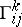, defined by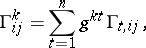where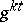is defined as follows: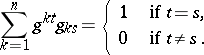These symbols were introduced by E.B. Christoffel in 1869.

#### Comments

Let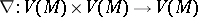,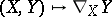, be a linear connection on a manifold, wheredenotes the space of vector fields on. Let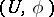be a chart of. Then on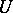,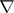is completely determined by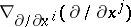, where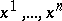are coordinates on. The Christoffel symbols of the connectionare now given byIt is important to note that theare not the components of a tensor field. In fact if the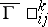denote the Christoffel symbols ofwith respect to a second set of coordinates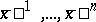on, thenLet nowbe the Riemannian connection (cf. Riemannian geometry) defined by a (local) Riemannian metric. Then the Christoffel symbols of this quadratic differential form are those of the connection. I.e.,so that indeed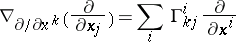where the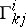are the Christoffel symbols of the second kind of the quadratic differential form as defined above.

How to Cite This Entry:
Christoffel symbol. Encyclopedia of Mathematics. URL: http://encyclopediaofmath.org/index.php?title=Christoffel_symbol&oldid=18161